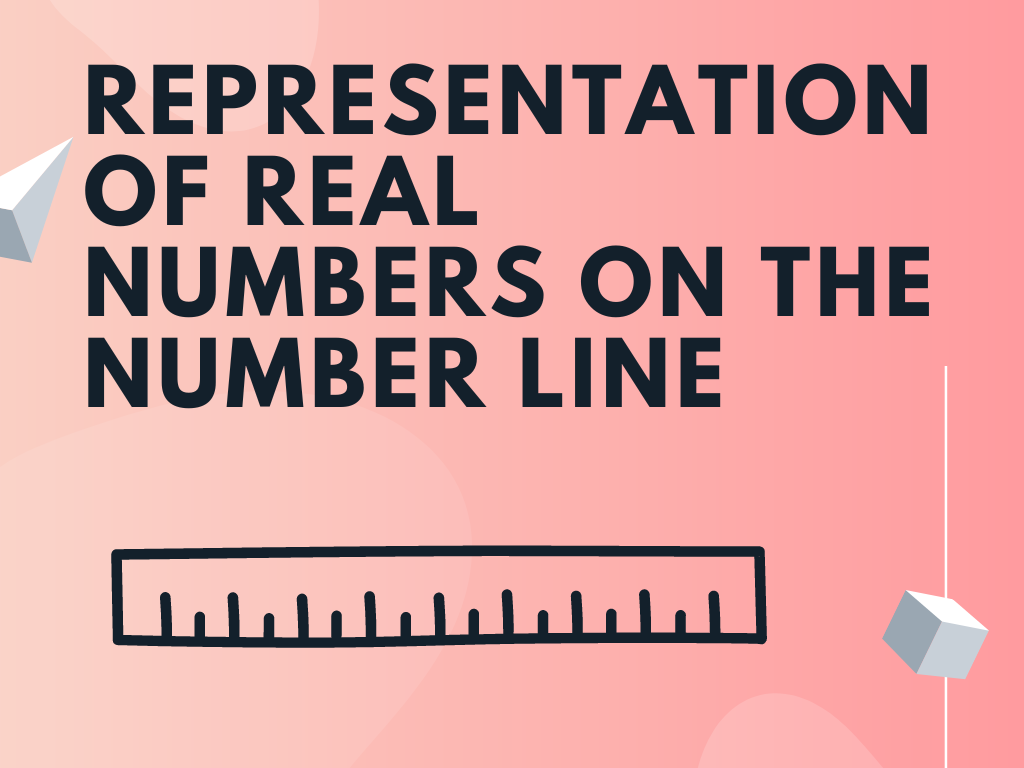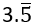# Representation of Real Numbers on the Number Line Class 9th

As per the knowledge of the previous classes, we are familiar with the representation of different types of numbers on the number line like the representation of natural numbers, whole numbers, integers, and rational numbers. In this section, we’ll learn the representation of real numbers on the number line including irrational numbers also. We’ll represent the real numbers when their decimal expansion is given.

We visualize the representation of numbers on the number line, through a magnifying glass. This method is known as the process of successive magnification.

### When the Decimal Expansion is Terminating

If the decimal expansion of a real number is terminating then how will we represent that number on the number line? Let us understand with the help of an example.

Example – Visualise the representation of 4.267 on the number line, using successive magnification.

Solution – Step I – First of all, we’ll find out between which two numbers the given number lies. The given number is 4.267 and it lies between 4 and 5.

Step II – After that, we’ll look closely at the portion on the number line between 4 and 5 with the help of magnifying glass. We know that there are 10 equal parts between any two numbers on the number line. We have 10 equal parts between 4 and 5. The first mark on the right of the number 4 represents 4.1, the second 4.2, and so on.

Step III – The number is 4.267 and we know that this will lie between 4.2 and 4.3. Now, we’ll focus on the portion between 4.2 and 4.3 and there are again 10 equal parts. The first mark represents 4.21, the second 4.22, and so on. With the help of magnifying glass, we can see it clearly.

Step IV – Again, the number 4.267 will lie between 4.26 and 4.27. There are again 10 equal parts between 4.26 and 4.27. The first mark represents 4.261, the second 4.262, and so on. So, the seventh mark in these subdivisions is 4.267 and this is our required number. We can see it large and clear after focusing on it with the help of magnifying glass.

The above process is the representation of a real number with a terminating decimal expansion on the number line.

### When the Decimal Expansion is Non-terminating Recurring

Let us understand with the help of an example, the representation of a real number when its decimal expansion is non-terminating recurring.

Example – Using successive magnification, visualize the representation ofon the number line, up to 4 decimal places.

Solution – We have to visualizeup to 4 decimal places, that is 3.5555.

Step I – At first, we know that the given number 3.5555 lies between the two numbers 3 and 4.

Step II – After that, if we look closer with the help of magnifying glass, at the portion of the number line, between 3 and 4, then we see that there are 10 equal parts between 3 and 4. The first mark on the right of the number 3 represents 3.1, the second 3.2, and so on.

Step III – The given number is 3.5555 and this will lie between 3.5 and 3.6. Now, we focus on the portion between 3.5 and 3.6. There are again 10 equal parts. The first mark represents 3.51, the second 3.52, and so on. We can see it large and clear with the help of magnifying glass.

Step IV – Again, the number 3.5555 will lie between 3.55 and 3.56. There are again 10 equal parts between 3.55 and 3.56. The first mark represents 3.551, the second 3.552, and so on.

Step V – Again, the number 3.5555 will lie between 3.555 and 3.556. We’ll focus between these numbers with the help of magnifying glass and we see there are again 10 equal parts. The first mark represents 3.5551, the second 3.5552, and so on. The fifth mark represents the number 3.5555 and this is our required number.

The above process is the representation of a real number with non-terminating recurring decimal expansion on the number line.

### When the Decimal Expansion is Non-terminating Non-Recurring

Let us understand with the help of an example, the representation of a real number when its decimal expansion is non-terminating non-recurring.

Example – Using successive magnification, visualize the representation of √2 = 1.414213562373095… on the number line, up to 3 decimal places, that is, up to 1.414.

Solution – Step I – We know the number 1.414 lies between the two numbers 1 and 2.

Step II – After this, we look closer at the portion between 1 and 2 with the help of magnifying glass. We can see there are 10 equal parts between 1 and 2. The first mark on the right of the number 1 represents 1.1, the second 1.2, and so on.

Step III – Our number 1.414 will lie between 1.4 and 1.5. We focus on the portion between 1.4 and 1.5 and there are again 10 equal parts. The first mark represents 1.41, the second 1.42, and so on.

Step IV – Again, the number 1.414 will lie between 1.41 and 1.42. There are again 10 equal parts between 1.41 and 1.42. The first mark represents 1.411, the second 1.412, and so on. The fourth mark represents 1.414 and this is our desired number.

The above process is the representation of a real number with non-terminating non-recurring decimal expansion on the number line.

Representation of √2 and √3 on the number line

Note – After solving the above examples, we can say that every real number is represented by a unique point on the number line and every point on the number line represents one and only one real number.

Representation of Real Numbers on the Number Line Class 9th in Hindi

Rate this post
Scroll to Top